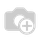# Tax calculation Problem in Purchase order Screen

hi all,

when I apply Tax for Purchase order Product.It display wrong calculation.

I have applied Excise 12% + VAT 5%.

Example:

Product Sub total is 1000

Excise 12 %

Vat      5 %

Total Tax  Value  :  173.60 correct value(179.78)

Amount With Tax:1173.60  correct value(1179.78)

How can I solve This Problem.```Hi zura,

As per my Indian accounting rules. I have apply 1st excise tax + 2nd.
service tax.

Let's take one example.
Price subtotal is 1000.
Excise is 12.50 %
Service tax is 5%.

As per the Indian accounting  rule first 12.50 is apply on 1000.
So,
1000*0.1250=125
1000+125=1125

now service tax is apply 5%. 1125 * 0.05=56.25

1000+125+56.25=1181.25
Total Tax=125+56.25=181.25
Total With Tax= 1181.25

But Openerp Display Wrong Fig.
Total Tax=175
Total With Tax=1000+175=1175

```Hi Hiral,

for me to improve my knowledge.
thanks dear.

On 5/21/15, Jeel Khanpara <jbk261292@gmail.com> wrote:
> hi,
>
> configuration for Tax is totally right as you suggest.
>

--
*JEEL B. KHANPARA*```

You configuration for Tax seems wrong.

Try with following:

Tax Name = Excise

Tax Type = Percentage

Value = 0.12500

Included in base amount = True

Tax Name = Vat

Tax Type = Percentage

Value = 0.05000

Included in base amount = FalseWhat I did was:

I noticed that I had Tax Computation under Taxes set as Percentage of Price Tax Included. I changed it to Percentage of Price and all came back to normal. I hope this works for you?